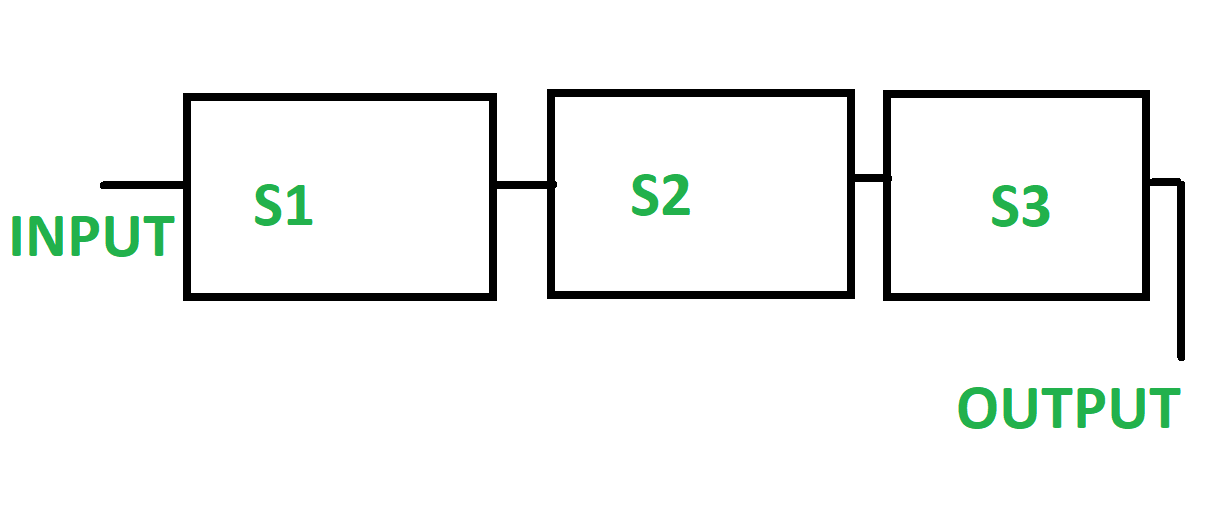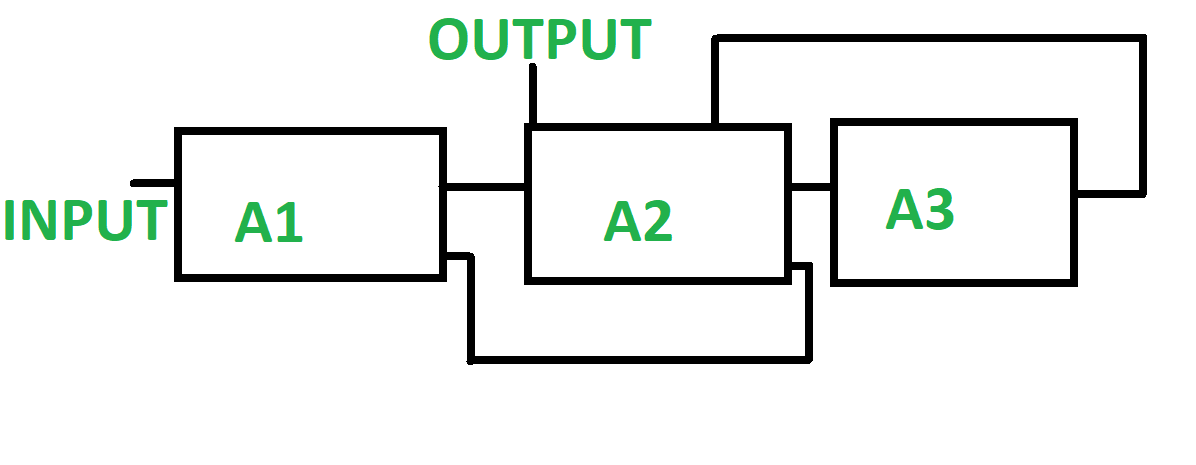Open In App

# Difference between Linear Pipeline and Non-Linear Pipeline

1. Linear Pipeline : Linear pipeline is a pipeline in which a series of processors are connected together in a serial manner. In linear pipeline the data flows from the first block to the final block of processor. The processing of data is done in a linear and sequential manner. The input is supplied to the first block and we get the output from the last block till which the processing of data is being done. The linear pipelines can be further be divided into synchronous and asynchronous models. Linear pipelines are typically used when the data transformation process is straightforward and can be performed in a single path.2. Non-Linear Pipeline : Non-Linear pipeline is a pipeline which is made of different pipelines that are present at different stages. The different pipelines are connected to perform multiple functions. It also has feedback and feed-forward connections. It is made such that it performs various function at different time intervals. In Non-Linear pipeline the functions are dynamically assigned. In Non-Linear pipeline the functions are dynamically assigned.Difference between Linear Pipeline and Non-Linear Pipeline :

Here’s an example code that demonstrates the difference between a linear pipeline and a non-linear pipeline. In this example, we’ll use the scikit-learn library in Python to build and compare the two pipeline types using a classification problem.

First, let’s start by installing the required libraries:

## Python

 `!pip install scikit``-``learn`

Now, let’s import the necessary modules and generate some synthetic data for classification:

## Python

 `from` `sklearn.datasets ``import` `make_classification``from` `sklearn.model_selection ``import` `train_test_split``from` `sklearn.linear_model ``import` `LogisticRegression``from` `sklearn.tree ``import` `DecisionTreeClassifier``from` `sklearn.pipeline ``import` `Pipeline``from` `sklearn.metrics ``import` `accuracy_score` `# Generate synthetic data for classification``X, y ``=` `make_classification(n_samples``=``1000``, n_features``=``10``, random_state``=``42``)` `# Split the data into training and testing sets``X_train, X_test, y_train, y_test ``=` `train_test_split(X, y, test_size``=``0.2``, random_state``=``42``)`

Now, let’s define and train a linear pipeline:

## Python

 `# Define a linear pipeline with logistic regression``linear_pipeline ``=` `Pipeline([``    ``(``'classifier'``, LogisticRegression())``])` `# Train the linear pipeline``linear_pipeline.fit(X_train, y_train)` `# Predict using the linear pipeline``linear_predictions ``=` `linear_pipeline.predict(X_test)` `# Calculate accuracy of the linear pipeline``linear_accuracy ``=` `accuracy_score(y_test, linear_predictions)``print``(``"Linear Pipeline Accuracy:"``, linear_accuracy)`

Next, let’s define and train a non-linear pipeline:

## Python

 `# Define a non-linear pipeline with decision tree classifier``nonlinear_pipeline ``=` `Pipeline([``    ``(``'classifier'``, DecisionTreeClassifier())``])` `# Train the non-linear pipeline``nonlinear_pipeline.fit(X_train, y_train)` `# Predict using the non-linear pipeline``nonlinear_predictions ``=` `nonlinear_pipeline.predict(X_test)` `# Calculate accuracy of the non-linear pipeline``nonlinear_accuracy ``=` `accuracy_score(y_test, nonlinear_predictions)``print``(``"Non-linear Pipeline Accuracy:"``, nonlinear_accuracy)`

In this code, we create a synthetic classification dataset using ‘make_classification‘ function from scikit-learn. We then split the data into training and testing sets using ‘train_test_split‘.

We define two pipelines, ‘linear_pipeline‘ and ‘nonlinear_pipeline‘. The linear pipeline consists of a single step, which is a logistic regression classifier. The non-linear pipeline consists of a single step, which is a decision tree classifier. Both pipelines are trained using the training data.

After training the pipelines, we make predictions on the testing data and calculate the accuracy of each pipeline using ‘accuracy_score‘. Finally, we print the accuracies of the linear and non-linear pipelines.

Output :

```Linear Pipeline Accuracy: 0.85
Non-linear Pipeline Accuracy: 0.82
```

In this example, the linear pipeline (using logistic regression) achieves an accuracy of 0.85 on the test data, while the non-linear pipeline (using a decision tree classifier) achieves an accuracy of 0.82. This shows that the linear pipeline performs slightly better than the non-linear pipeline for this particular dataset and problem. However, the actual values may differ depending on the random data generation and the specific characteristics of the dataset being used.